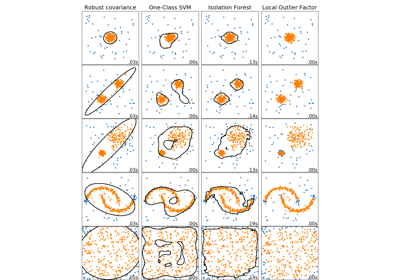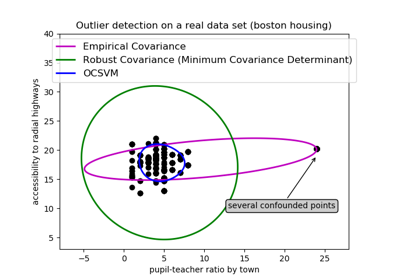# sklearn.covariance.EllipticEnvelope¶

class sklearn.covariance.EllipticEnvelope(store_precision=True, assume_centered=False, support_fraction=None, contamination=0.1, random_state=None)[source]

An object for detecting outliers in a Gaussian distributed dataset.

Read more in the User Guide.

Parameters
store_precisionboolean, optional (default=True)

Specify if the estimated precision is stored.

assume_centeredboolean, optional (default=False)

If True, the support of robust location and covariance estimates is computed, and a covariance estimate is recomputed from it, without centering the data. Useful to work with data whose mean is significantly equal to zero but is not exactly zero. If False, the robust location and covariance are directly computed with the FastMCD algorithm without additional treatment.

support_fractionfloat in (0., 1.), optional (default=None)

The proportion of points to be included in the support of the raw MCD estimate. If None, the minimum value of support_fraction will be used within the algorithm: [n_sample + n_features + 1] / 2.

contaminationfloat in (0., 0.5), optional (default=0.1)

The amount of contamination of the data set, i.e. the proportion of outliers in the data set.

random_stateint, RandomState instance or None, optional (default=None)

The seed of the pseudo random number generator to use when shuffling the data. If int, random_state is the seed used by the random number generator; If RandomState instance, random_state is the random number generator; If None, the random number generator is the RandomState instance used by np.random.

Attributes
location_array-like, shape (n_features,)

Estimated robust location

covariance_array-like, shape (n_features, n_features)

Estimated robust covariance matrix

precision_array-like, shape (n_features, n_features)

Estimated pseudo inverse matrix. (stored only if store_precision is True)

support_array-like, shape (n_samples,)

A mask of the observations that have been used to compute the robust estimates of location and shape.

offset_float

Offset used to define the decision function from the raw scores. We have the relation: decision_function = score_samples - offset_. The offset depends on the contamination parameter and is defined in such a way we obtain the expected number of outliers (samples with decision function < 0) in training.

Notes

Outlier detection from covariance estimation may break or not perform well in high-dimensional settings. In particular, one will always take care to work with n_samples > n_features ** 2.

References

R68ae096da0e4-1

Rousseeuw, P.J., Van Driessen, K. “A fast algorithm for the minimum covariance determinant estimator” Technometrics 41(3), 212 (1999)

Examples

>>> import numpy as np
>>> from sklearn.covariance import EllipticEnvelope
>>> true_cov = np.array([[.8, .3],
...                      [.3, .4]])
>>> X = np.random.RandomState(0).multivariate_normal(mean=[0, 0],
...                                                  cov=true_cov,
...                                                  size=500)
>>> cov = EllipticEnvelope(random_state=0).fit(X)
>>> # predict returns 1 for an inlier and -1 for an outlier
>>> cov.predict([[0, 0],
...              [3, 3]])
array([ 1, -1])
>>> cov.covariance_
array([[0.7411..., 0.2535...],
[0.2535..., 0.3053...]])
>>> cov.location_
array([0.0813... , 0.0427...])


Methods

 correct_covariance(self, data) Apply a correction to raw Minimum Covariance Determinant estimates. decision_function(self, X) Compute the decision function of the given observations. error_norm(self, comp_cov[, norm, scaling, …]) Computes the Mean Squared Error between two covariance estimators. fit(self, X[, y]) Fit the EllipticEnvelope model. fit_predict(self, X[, y]) Perform fit on X and returns labels for X. get_params(self[, deep]) Get parameters for this estimator. get_precision(self) Getter for the precision matrix. mahalanobis(self, X) Computes the squared Mahalanobis distances of given observations. predict(self, X) Predict the labels (1 inlier, -1 outlier) of X according to the fitted model. reweight_covariance(self, data) Re-weight raw Minimum Covariance Determinant estimates. score(self, X, y[, sample_weight]) Returns the mean accuracy on the given test data and labels. score_samples(self, X) Compute the negative Mahalanobis distances. set_params(self, \*\*params) Set the parameters of this estimator.
__init__(self, store_precision=True, assume_centered=False, support_fraction=None, contamination=0.1, random_state=None)[source]

Initialize self. See help(type(self)) for accurate signature.

correct_covariance(self, data)[source]

Apply a correction to raw Minimum Covariance Determinant estimates.

Correction using the empirical correction factor suggested by Rousseeuw and Van Driessen in [RVD].

Parameters
dataarray-like, shape (n_samples, n_features)

The data matrix, with p features and n samples. The data set must be the one which was used to compute the raw estimates.

Returns
covariance_correctedarray-like, shape (n_features, n_features)

Corrected robust covariance estimate.

References

RVD

A Fast Algorithm for the Minimum Covariance Determinant Estimator, 1999, American Statistical Association and the American Society for Quality, TECHNOMETRICS

decision_function(self, X)[source]

Compute the decision function of the given observations.

Parameters
Xarray-like, shape (n_samples, n_features)
Returns
decisionarray-like, shape (n_samples, )

Decision function of the samples. It is equal to the shifted Mahalanobis distances. The threshold for being an outlier is 0, which ensures a compatibility with other outlier detection algorithms.

error_norm(self, comp_cov, norm='frobenius', scaling=True, squared=True)[source]

Computes the Mean Squared Error between two covariance estimators. (In the sense of the Frobenius norm).

Parameters
comp_covarray-like of shape (n_features, n_features)

The covariance to compare with.

normstr

The type of norm used to compute the error. Available error types: - ‘frobenius’ (default): sqrt(tr(A^t.A)) - ‘spectral’: sqrt(max(eigenvalues(A^t.A)) where A is the error (comp_cov - self.covariance_).

scalingbool

If True (default), the squared error norm is divided by n_features. If False, the squared error norm is not rescaled.

squaredbool

Whether to compute the squared error norm or the error norm. If True (default), the squared error norm is returned. If False, the error norm is returned.

Returns
The Mean Squared Error (in the sense of the Frobenius norm) between
self and comp_cov covariance estimators.
fit(self, X, y=None)[source]

Fit the EllipticEnvelope model.

Parameters
Xnumpy array or sparse matrix, shape (n_samples, n_features).

Training data

yIgnored

not used, present for API consistency by convention.

fit_predict(self, X, y=None)[source]

Perform fit on X and returns labels for X.

Returns -1 for outliers and 1 for inliers.

Parameters
Xndarray, shape (n_samples, n_features)

Input data.

yIgnored

Not used, present for API consistency by convention.

Returns
yndarray, shape (n_samples,)

1 for inliers, -1 for outliers.

get_params(self, deep=True)[source]

Get parameters for this estimator.

Parameters
deepbool, default=True

If True, will return the parameters for this estimator and contained subobjects that are estimators.

Returns
paramsmapping of string to any

Parameter names mapped to their values.

get_precision(self)[source]

Getter for the precision matrix.

Returns
precision_array-like

The precision matrix associated to the current covariance object.

mahalanobis(self, X)[source]

Computes the squared Mahalanobis distances of given observations.

Parameters
Xarray-like of shape (n_samples, n_features)

The observations, the Mahalanobis distances of the which we compute. Observations are assumed to be drawn from the same distribution than the data used in fit.

Returns
distarray, shape = [n_samples,]

Squared Mahalanobis distances of the observations.

predict(self, X)[source]

Predict the labels (1 inlier, -1 outlier) of X according to the fitted model.

Parameters
Xarray-like, shape (n_samples, n_features)
Returns
is_inlierarray, shape (n_samples,)

Returns -1 for anomalies/outliers and +1 for inliers.

reweight_covariance(self, data)[source]

Re-weight raw Minimum Covariance Determinant estimates.

Re-weight observations using Rousseeuw’s method (equivalent to deleting outlying observations from the data set before computing location and covariance estimates) described in [RVDriessen].

Parameters
dataarray-like, shape (n_samples, n_features)

The data matrix, with p features and n samples. The data set must be the one which was used to compute the raw estimates.

Returns
location_reweightedarray-like, shape (n_features, )

Re-weighted robust location estimate.

covariance_reweightedarray-like, shape (n_features, n_features)

Re-weighted robust covariance estimate.

support_reweightedarray-like, type boolean, shape (n_samples,)

A mask of the observations that have been used to compute the re-weighted robust location and covariance estimates.

References

RVDriessen

A Fast Algorithm for the Minimum Covariance Determinant Estimator, 1999, American Statistical Association and the American Society for Quality, TECHNOMETRICS

score(self, X, y, sample_weight=None)[source]

Returns the mean accuracy on the given test data and labels.

In multi-label classification, this is the subset accuracy which is a harsh metric since you require for each sample that each label set be correctly predicted.

Parameters
Xarray-like, shape (n_samples, n_features)

Test samples.

yarray-like, shape (n_samples,) or (n_samples, n_outputs)

True labels for X.

sample_weightarray-like, shape (n_samples,), optional

Sample weights.

Returns
scorefloat

Mean accuracy of self.predict(X) wrt. y.

score_samples(self, X)[source]

Compute the negative Mahalanobis distances.

Parameters
Xarray-like, shape (n_samples, n_features)
Returns
negative_mahal_distancesarray-like, shape (n_samples, )

Opposite of the Mahalanobis distances.

set_params(self, **params)[source]

Set the parameters of this estimator.

The method works on simple estimators as well as on nested objects (such as pipelines). The latter have parameters of the form <component>__<parameter> so that it’s possible to update each component of a nested object.

Parameters
**paramsdict

Estimator parameters.

Returns
selfobject

Estimator instance.

## Examples using sklearn.covariance.EllipticEnvelope¶